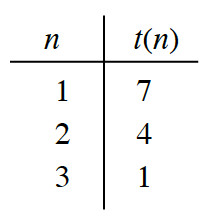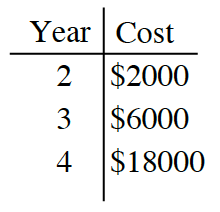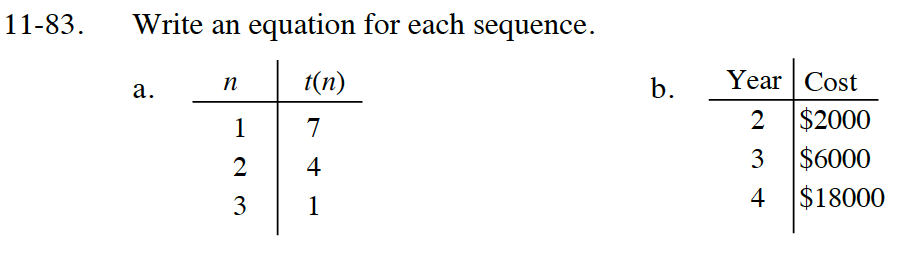Home > CC4 > Chapter 11 > Lesson 11.2.3 > Problem11-83

11-83.
1. Write an equation for each sequence. Homework Help ✎

2.  a.b.Review the Math Notes box in section 2.2.2 and section 5.3.3.

Do these points match an Arithmetic sequence or Geometric sequence?